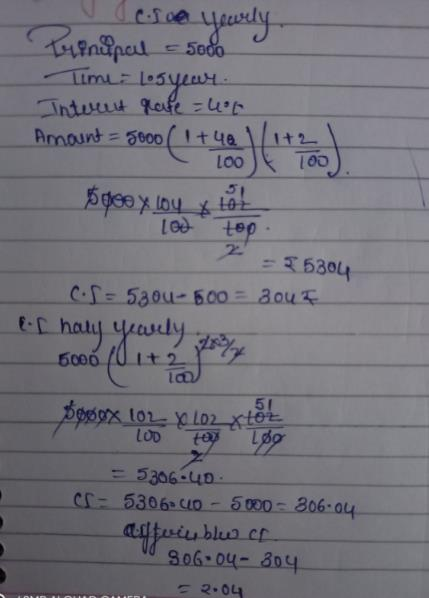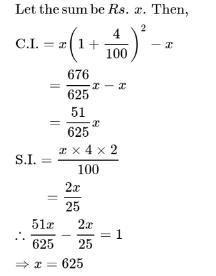Courses

# Test: Compound Interest- 1

## 10 Questions MCQ Test General Aptitude for GATE 2020 | Test: Compound Interest- 1

Description
This mock test of Test: Compound Interest- 1 for Quant helps you for every Quant entrance exam. This contains 10 Multiple Choice Questions for Quant Test: Compound Interest- 1 (mcq) to study with solutions a complete question bank. The solved questions answers in this Test: Compound Interest- 1 quiz give you a good mix of easy questions and tough questions. Quant students definitely take this Test: Compound Interest- 1 exercise for a better result in the exam. You can find other Test: Compound Interest- 1 extra questions, long questions & short questions for Quant on EduRev as well by searching above.
QUESTION: 1

### What will be the compound interest on a sum of Rs. 40,000 after 3 years at the rate of 11 p.c.p.a.?

Solution:

Amount after 3 years = P(1 + R/100)T
=> 40000(1 + 11/100)3
=> 40000(111/100)3
=> 40000[(111*111*111)/(100*100*100)]
=> (4*111*111*111)/100
=> 54705.24
Compound Interest = 54705.24 - 40000
= Rs. 14705.24

QUESTION: 2

Solution:
QUESTION: 3

### Q. What is the difference between the compound interests on Rs. 5000 for 11/2  years at 4 percent per annum compounded yearly and half yearly?

Solution:QUESTION: 4

If the simple interest on a sum of money for 2 years at 5% per annum is Rs. 60, what is the compound interest on the same at the same rate and for the same time?

Solution:
QUESTION: 5

There is 80% increase in an amount in 8 years at simple interest. What will be the compound interest of Rs. 14,000 after 3 years at the same rate?

Solution:

Simple interest = P * r * t /100, where, P is the Principal, r is the rate of interest and t is the time period such that the rate of interest and the time period have mutually compatible units like r % per annum and t years.

Here, in the first case, let the Principal be Rs.P and rate of interest be r % per annum. Time period is given as 8 years . Interest is given as 80 P / 100
So, 80 P / 100 = P * r * 8 / 100
Or, 80 = 8 r
So, r = 10 % per annum.

Now, in the second case, Principal is given as Rs. 14,000, rate of compound interest is 10 % per annum as determined above; and t is 3 years.
Compound interest = P * ( 1 + r/100)t - P
= 14,000 * ( 1+ 10 /100)3 - 14,000
= 14,000 * ( 1 + 0.1)3 - 14,000
= 14,000 *(1.1)3 - 14,000
(14,000 * 1.331) - 14,000
= 18,634 - 14,000 = 4,634
So, the compound interest on Rs.14,000 at the same rate of interest as in case 1 in 3 years will be Rs. 4,634.

QUESTION: 6

The compound interest on Rs. 30,000 at 7% per annum is Rs. 4347. The period (in years) is:

Solution:

Amount = Rs.(30000+4347) = Rs.34347
let the time be n years
Then,30000(1+7/100)n = 34347
(107/100)n = 34347/30000
= 11449/10000
= (107/100)2
n = 2years

QUESTION: 7

At what rate of compound interest per annum will a sum of Rs. 1400 become Rs. 1573.04 in 2 years?

Solution:

Let the rate be R% per annum
P(1+R/100)T = 1573.04
= 1400(1+R/100)2 = 1573.04
= (1+R/100)2 = 1573.04/1400 = 157304/140000 = 11236/10000
(1+R/100) = (√11236/10000)
= (√11236)/(√10000)
= 106/100
R/100 = (106/100 − 1)
=> 6/100
R = 6%

QUESTION: 8

The difference between compound interest and simple interest on an amount of Rs. 15,000 for 2 years is Rs. 96. What is the rate of interest per annum?

Solution:

[15000×(1+R/100)2 − 15000] - (15000×R×2)/100 = 96
⇒15000[(1+R/100)2 − 1 − 2R/100]=96
⇒ 15000[(100+R)2 − 10000−(200×R)]/10000 = 96
⇒ R2 = (96×23) = 64
⇒ R = 8
∴ Rate = 8%

QUESTION: 9

The difference between simple interest and compound on Rs. 900 for one year at 10% per annum reckoned half-yearly is:

Solution:
QUESTION: 10

The difference between simple and compound interests compounded annually on a certain sum of money for 2 years at 4% per annum is Re. 1. The sum is:

Solution: